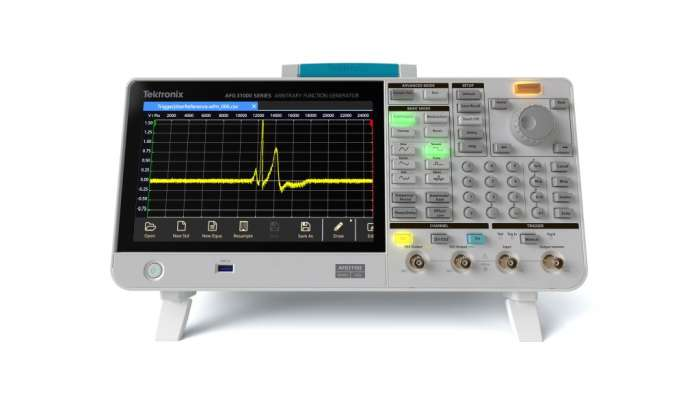# INTERFACING ADC 0804 with 8051 MICROCONTROLLER

INTERFACING ADC USING 8051 MICROCONTROLLER: In this article you will learn how to interface, analog to digital converter with 8051 microcontroller. Unlike, pic microcontrollerArduino and avr microcontroller, 8051 microcontroller do not have built in ADC.  if we want to interface any sensor with 8051 microcontroller, we have to use external ADC. For example, you want to measure temperature with 8051 microcontroller and you are using LM35 temperature sensor to measure temperature. LM35 temperature sensor gives output in the form of analog voltage. So we need to use analog to digital converter. We use Analogue to digital convertor (ADC) to convert the analogue signal into digital form. Analogue signal can be the output of some sensor. And then the data in digital format can then be used for further processing by the digital processors. Now in this tutorial we will learn about ADC 0804 and its interfacing using 8051 microcontroller.

FEATURES OF ADC 0804:

Its main features are given below:

• 8 bit resolution
• Differential analogue voltage inputs
• 0-5V input voltage range
• No zero adjustment
• Built-in clock generator
• Voltage at Vref/2 (pin9) can be externally adjusted to convert smaller input voltage spans to full 8 bit resolution.

RESOLUTION:

Resolution refers to the conversion of an analog voltage to a digital value. It indicates the number of discrete values that an ADC can produce over the range of analog values. The values are usually stored electronically in binary form, so the resolution is usually expressed in bits.

STEP SIZE:

It is the voltage difference between one digital level and the next level that can be measured by ADC. In a 4-bit converter, an input of 1 Volt produce an output of 0001, then the step size is 1 Volt. 0 volts is always considered 0000. Distortion in the signal can be reduced by decreasing the step size to 0.5 voltsbut it lowers the maximum input reading. Ife we are using smaller step size, higher bit converter is needed to have maximum voltage.

READ  A digital thermometer or talk I2C to your atmel microcontroller using pic microcontroller

ADC0804 is a single channel analog to digital convertor. It takes only one analog signal. It has 8 bit resolution. Other ADC can have n bit resolution and n can be 8,10,12,16 or 24 bits. If ADC has higher resolution, it gives smaller step size.If ADC has 8 bit resolution, input voltage span is 0-5V and the step size is 19.53mV (5V/255). In this situation we called Vref/2 as left open.

PIN DIAGRAM:PIN DESCRIPTION:

Pin description of ADC0804 is given below:

CS:                  Chip Select
It is an active low pin and is used to activate ADC0804

It is an input pin and is active low. ADC stores the result in an internal register after conversion of analog data. This pin helps to get the data out of the ADC0804.
When CS=0, high to low pulse is given to RD pin, then digital output comes on the pins D0-D7

WR:                Write
It is an input pin and is active low which is used to initiate the ADC to start the conversion process.
When CS=0, WR makes a low to high transition, then ADC starts the conversion process.

CLK IN:        Clock IN
This is an input pin which is connected to an external clock source.

INTR:             Interrupt
This is an output pin and is active low.When the conversion is over, this pin goes low.

AGND:           Analog Ground
Connected to ground.

Vref/2:            Reference Voltage
Used to set the reference voltage. Default reference voltage is 5V when not connected.Step size can be reduced by using this pin.

READ  PIC Microcontroller Video Tutorial Series

DGND:           Digital Ground
Connected to ground.

D7-D0:            Output Data Bits

Output bits of binary data.

CLKR:           Clock Reset
To reset the clock.

Vcc:                Positive Supply
Power supply of ADC.

CONVERSION STEPS:

• Firstly make CS=0.
• Send a low to high pulse to WR pin to start the conversion.
• Keep checking the INTR pin.

INTR = 1, if conversion is not finished, poll until it is finished

INTR = 0, if conversion is finished.

• If conversion is finished (INTR=0), Ensure CS=0
• Then, send a high to low pulse to RD pin to read the data from the ADC.
• If we connect CS of ADC to ground, then there will be no need to make or ensure it to zero.

CONNECTION TO 8051 MCU:

As we know that microcontroller willprovide control signals to the ADC. Its main connections are as follow:• CS pin of ADC is connected to ground.
• 5of MCU is connected to WR of ADC0804.
• 6 of MCU is connected to RD of ADC0804.
• 4 of MCU is connected to INTR of ADC0804.
• Output is demonstrated through LEDs which are connected between the Data out pins of ADC0804 and port 0 of 8051 microcontroller.
• Input voltage from the preset is varied and the output of ADC varies.

PROTEUS SIMULATION:WORKING:

Port 1 is used as input port and port 0 is used as output port. Potentiometer is used to adjust the voltage.  If the analogue input voltage is 5V then all LEDs will glow indicating 11111111 in binary which is the equivalent of 255 in decimal. If the voltage is zero, no LED will glow.

READ  Microchip PIC Serial Port Programmer

When full voltage given:(Output =11111111)When half voltage given:INTERFACING ADC 0804 with 8051 MICROCONTROLLER

#### Current Project / Post can also be found using:

• Adc pic assembly cs wr